# Integration Formulas

Integration Formulas

Let's recap a bit before diving into integration. You already have an intuitive idea of derivatives.

If we differentiate a function $$f$$ in an interval $$I$$, then we get a family of functions in $$I$$.

What if the values of functions are known in $$I$$?

Can you determine the function $$f$$?

Yes, we do it using the method of integration.

Basically, integration is a way of uniting the part to find a whole.

A pizza is integration and each slice of the pizza is the derivative.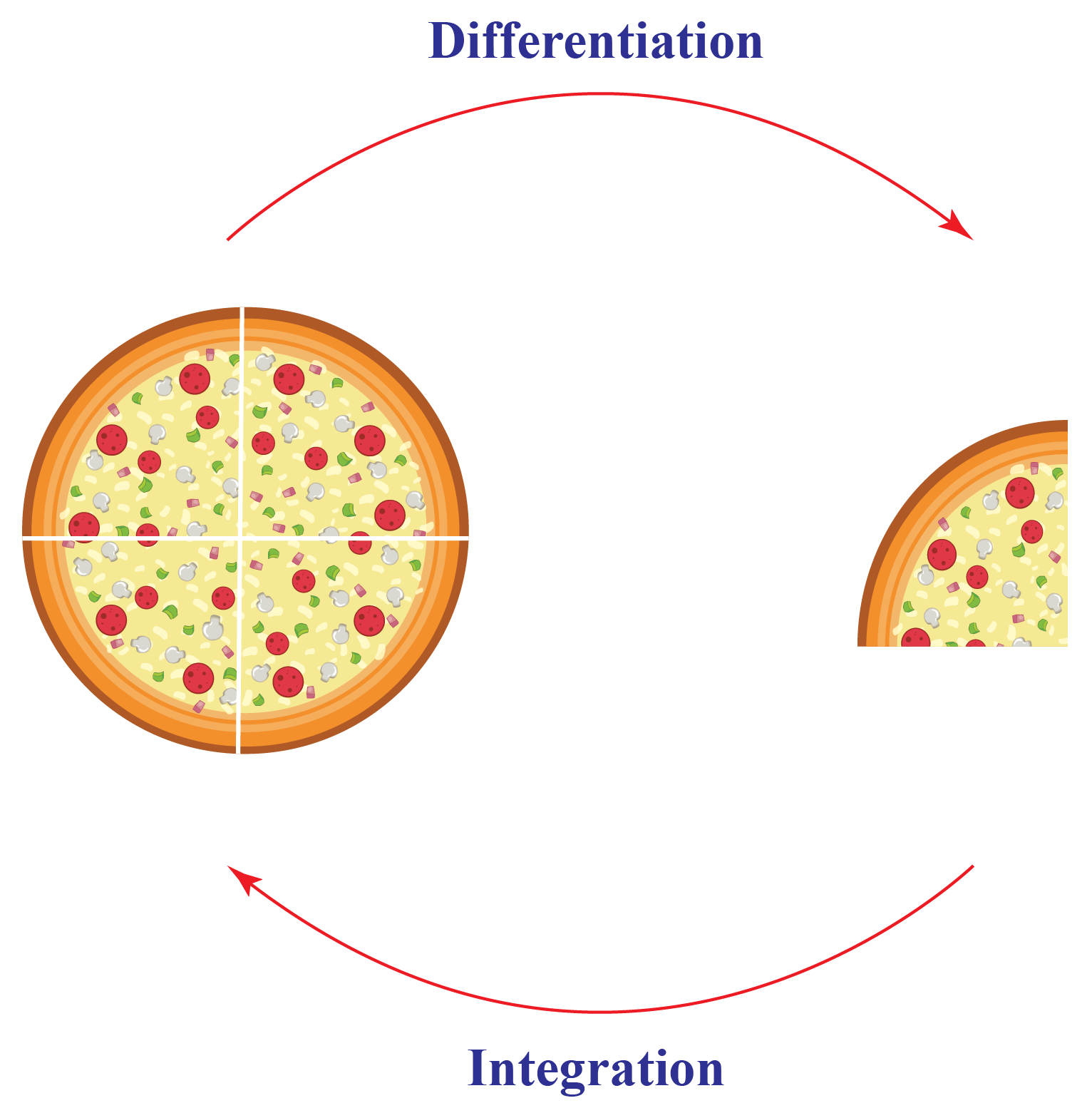Here, the heart of the matter is the integration of a function in its domain of definition.

Let's move further and learn about integration and some of its powerful techniques.

## Lesson Plan

 1 What is The Basic Formula of Integration? 2 Important Notes on Integration Formulas 3 Solved Examples on Integration Formulas 4 Challenging Questions on Integration Formulas

## What is The Basic Formula of Integration?

Given the derivative $$f’$$ of the function $$f$$, the natural question arises is can we determine the function$$f$$?

The functions that give the derivative, that is, the function $$f$$ is called antiderivative or integral of $$f’$$.

The process of finding the antiderivative is called integration.

For example, the derivative of $$f(x)=x^{3}$$ is $$f’(x)=3x^{2}$$ and the antiderivative of $$g(x)=3x^{2}$$ is $$f(x)=x^{3}$$.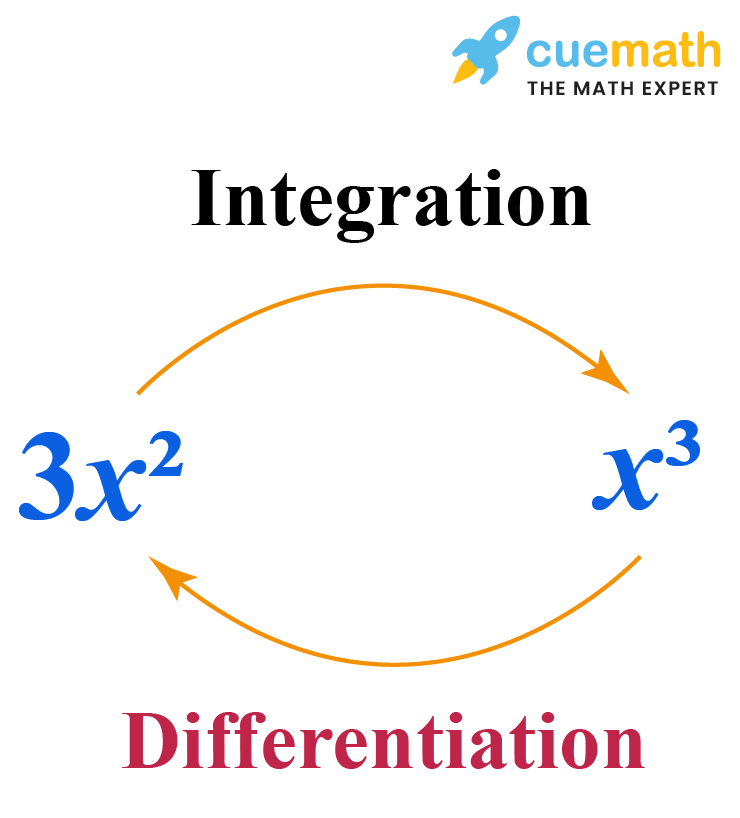## What are the Three Integration Methods?

There are three main methods to reduce the function in the standard form to find its integral.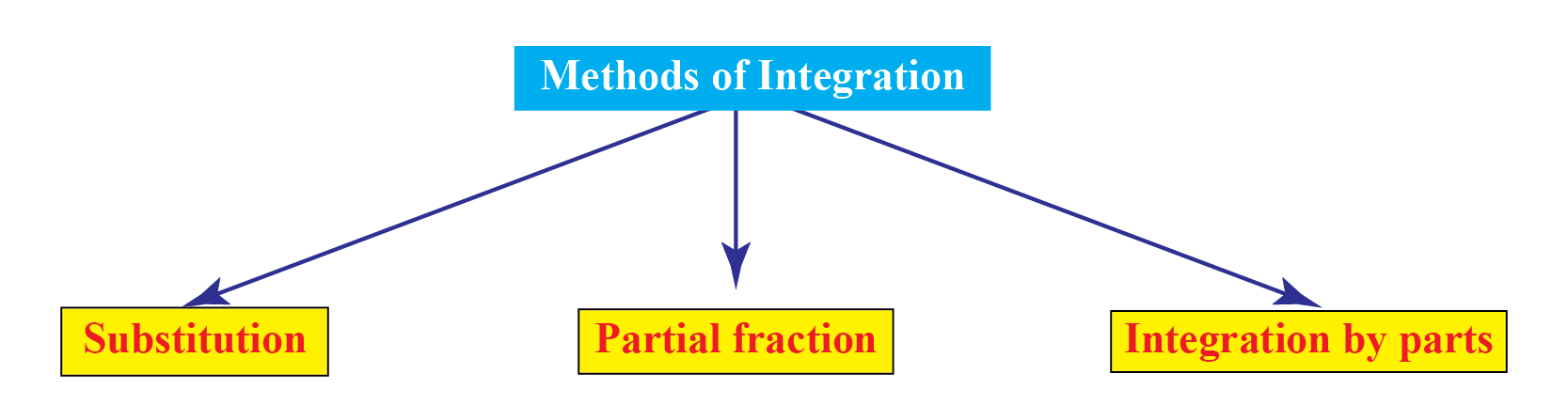These methods are given below.

### Method 1: Substitution

Suppose, we have to find $$y=\int f(x) dx$$.

Let $$x=g(t)$$. Then, $$\dfrac{dx}{dt}=g'(t)$$.

So, $$y=\int f(x) dx$$ can be written as $$y=\int f(g(t)) g'(t)dt$$.

For example, let's find the integral of $$f(x)=\sin{mx}$$ using substitution.

Let $$mx=t$$. Then, $$m\dfrac{dx}{dt}=1$$.

So,

\begin{align}y&=\int \sin{mx}dx\\&=\dfrac{1}{m}\int \sin{t}dt\\&=-\dfrac{1}{m} \cos{t}+C\\&=-\dfrac{1}{m} \cos{mx}+C\end{align}

$$y=\int \sin{mx}dx$$ can be written as $$y=\int f(g(t)) g'(t)dt$$.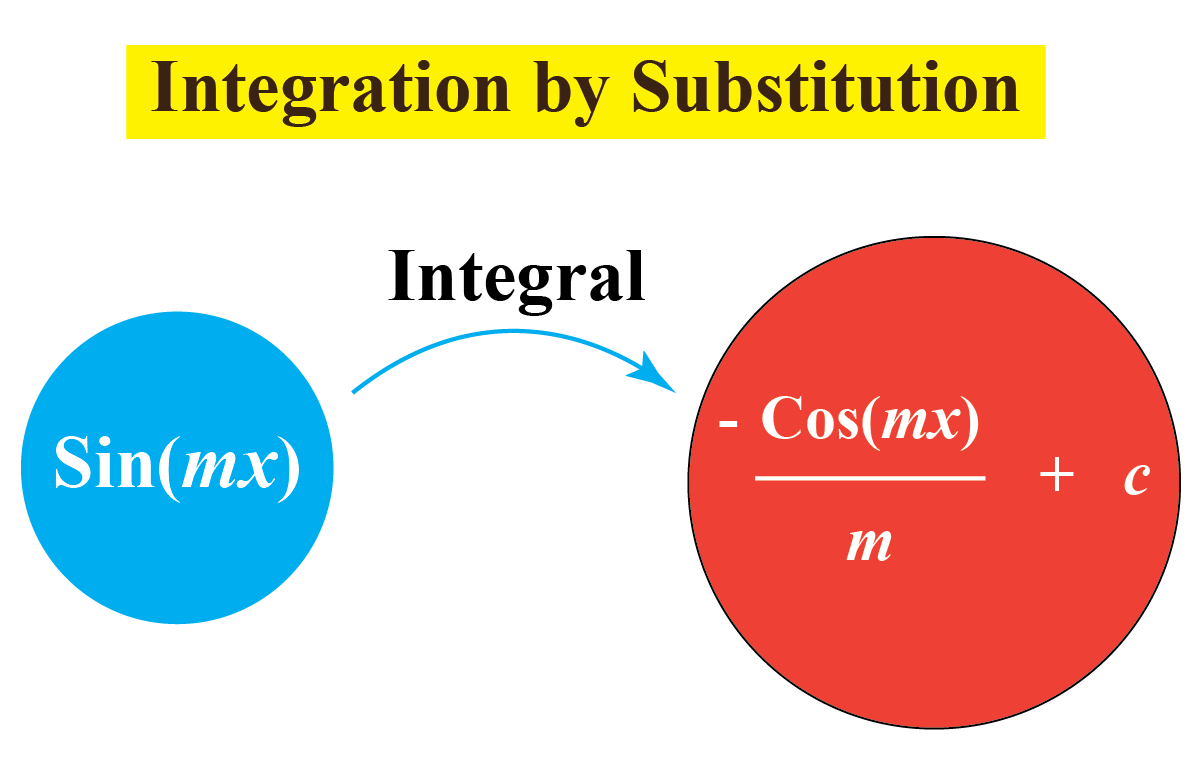### Method 2: Partial Fractions

Suppose we have to find $$y=\int \dfrac{P(x)}{Q(x)} dx$$ where $$\dfrac{P(x)}{Q(x)}$$ is an improper rational function.

We reduce it in such a way that $$\dfrac{P(x)}{Q(x)}=T(x)+\dfrac{P_{1}(x)}{Q(x)}$$.

Here, $$T(x)$$ is polynomial in $$x$$ and $$\dfrac{P_{1}(x)}{Q(x)}$$ is proper rational function.

The following table shows some rational function and their corresponding rational functions.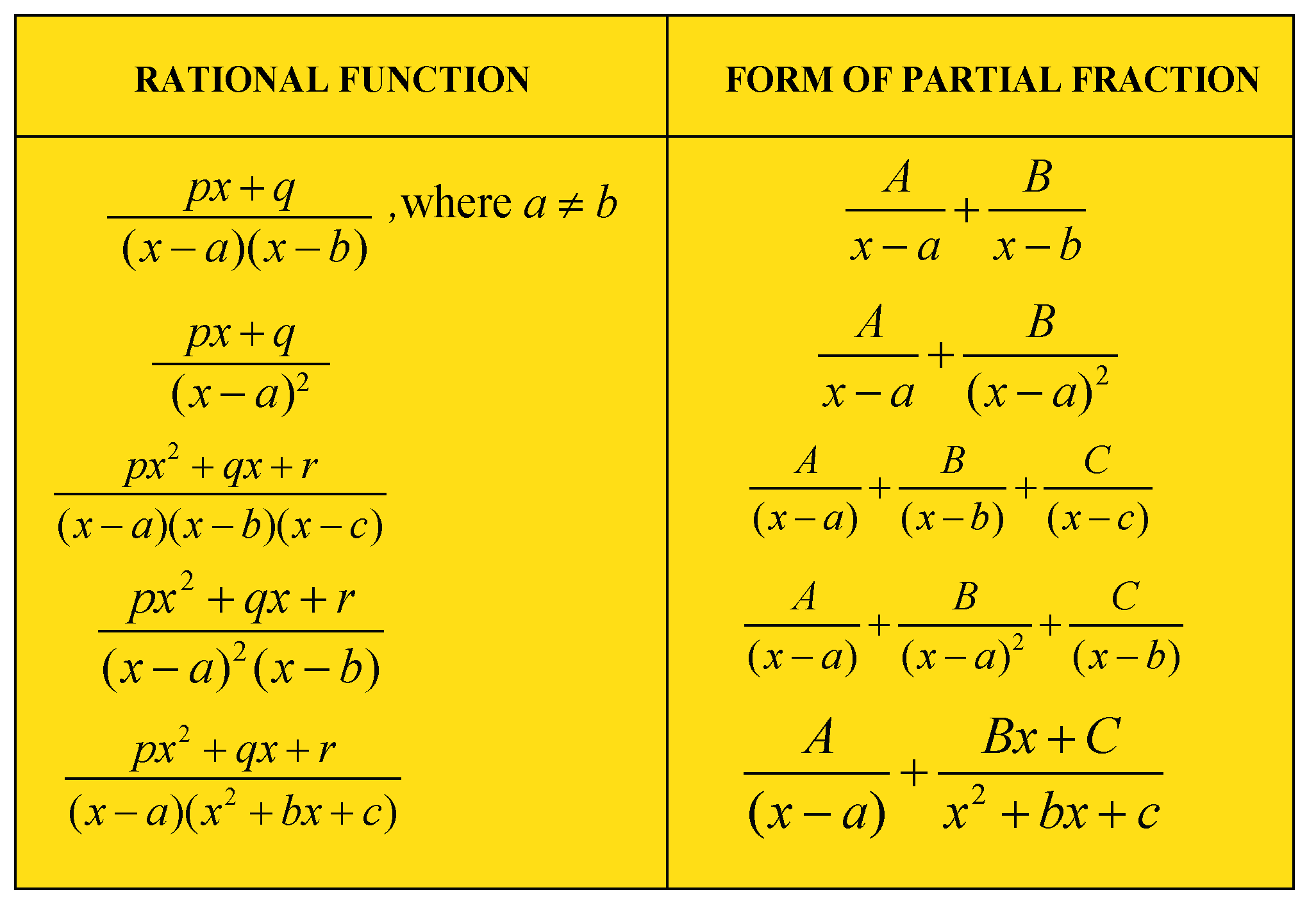Hence we have: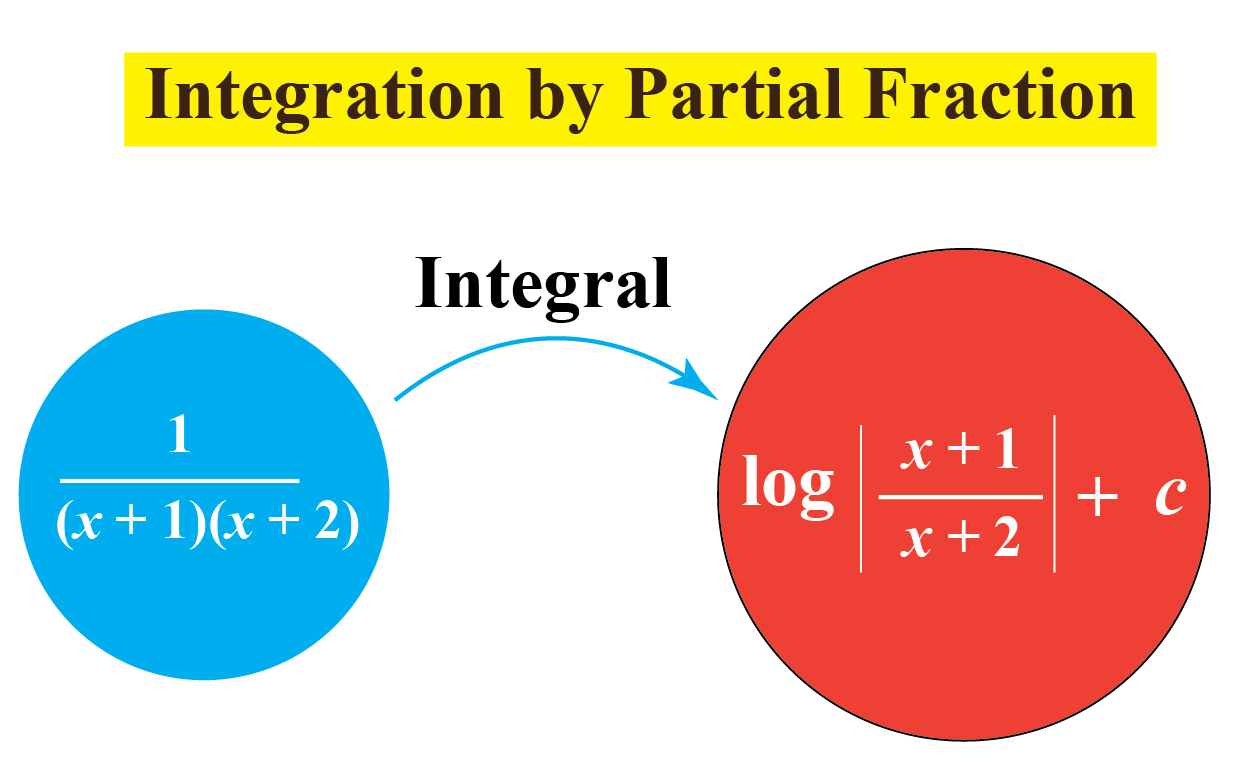### Method 3: Integration by Parts

This Integration rule is used to find the integral of two functions.

By product rule of derivatives, we have $$\dfrac{d}{dx}(uv)=u\dfrac{dv}{dx}+v\dfrac{du}{dx}\;\;\;\;\;\;\; \cdots (1)$$

Integration on both sides of equation (1), we get $$\int u\dfrac{dv}{dx}dx=uv-\int v\dfrac{du}{dx} dx\;\;\;\;\;\;\; \cdots (2)$$

Equation (2) can be written as $$uv=\int u\dfrac{dv}{dx}dx+\int v\dfrac{du}{dx} dx$$

Let $$u=f(x)$$ and $$\dfrac{dv}{dx}=g(x)$$.

Then, we have $$\dfrac{du}{dx}=f'(x)$$ and $$v=\int g(x)dx$$.

So, Equation (2) becomes \begin{align}\int f(x) g(x)dx\\=f(x) \int g(x)dx-\int [f'(x) \int g(x)dx]dx\end{align}

For example, let's find the integral of $$xe^{x}$$ using integration by parts.

\begin{align}\int xe^{x}dx&=x \int e^{x}dx-\int \left[\dfrac{dx}{dx}\int e^{x}dx\right]dx\\&=x e^{x}-\int [e^{x}]dx\\&=x e^{x}-e^{x}+C\end{align}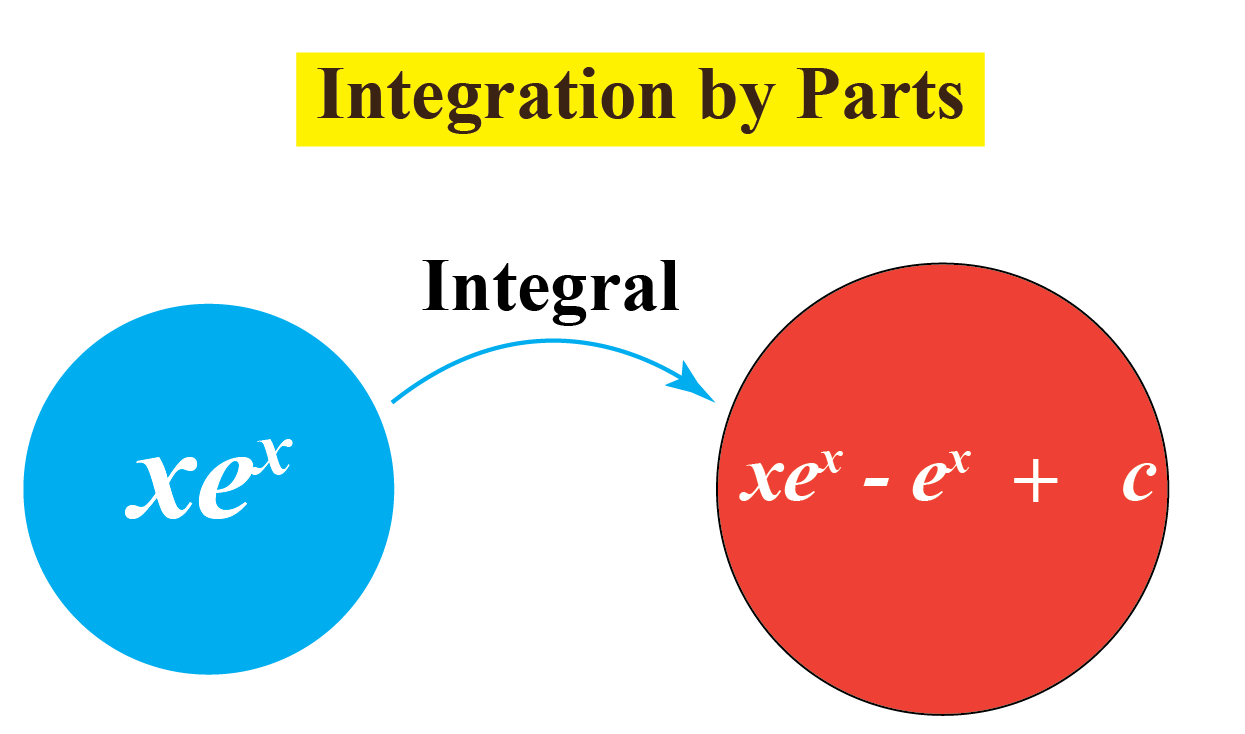## What Are The Different Types of Integration?

In general, there are two types of integrals.

### Definite Integration

These are the integrations that have a pre-existing value of limits; thus making the final value of integral definite.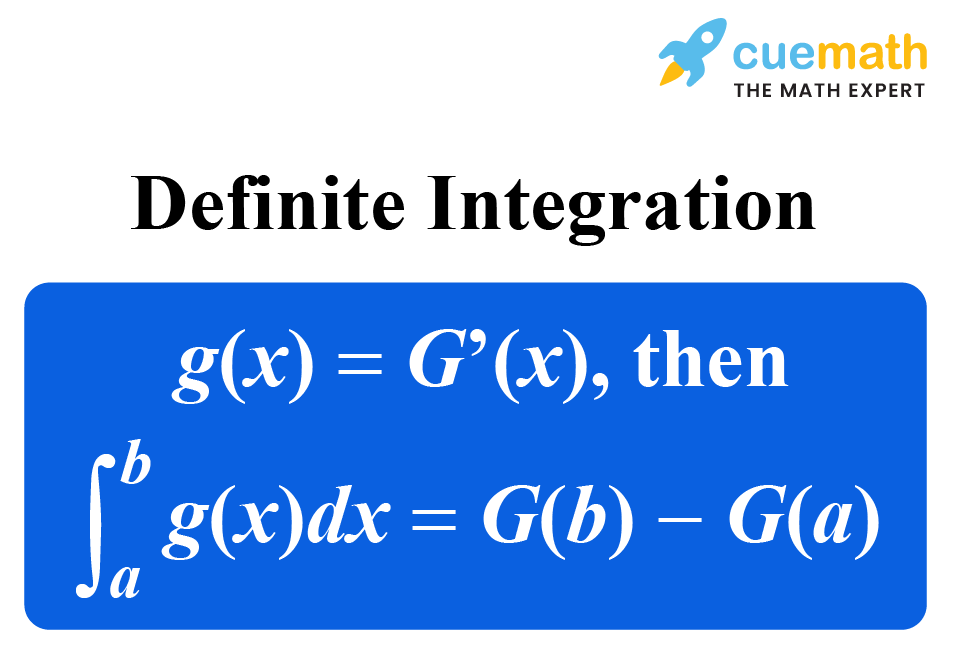### Indefinite Integration

These are the integrations that do not have a pre-existing value of limits; thus making the final value of integral indefinite.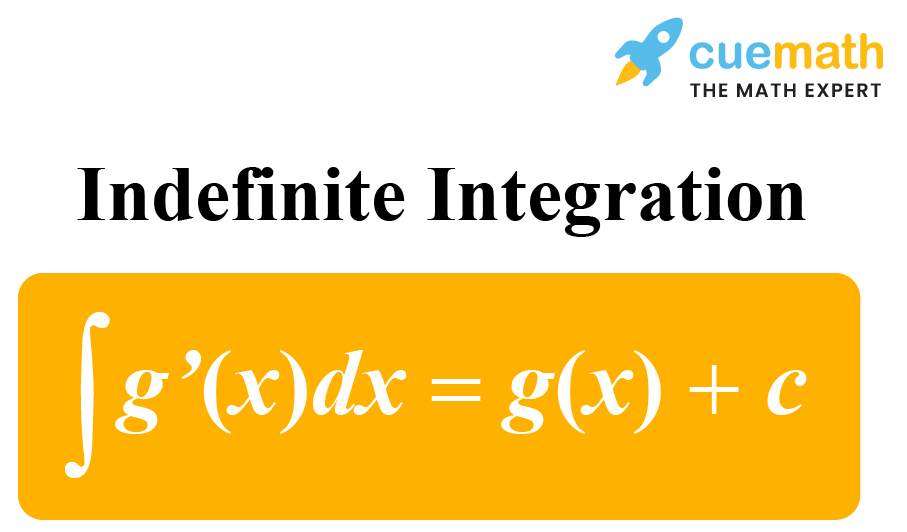Here, $$c$$ is the integration constant.

## What Are the Different Integration Formulas?

We already know the formulas of derivatives of some important functions.

Here are the corresponding integrals of these functions.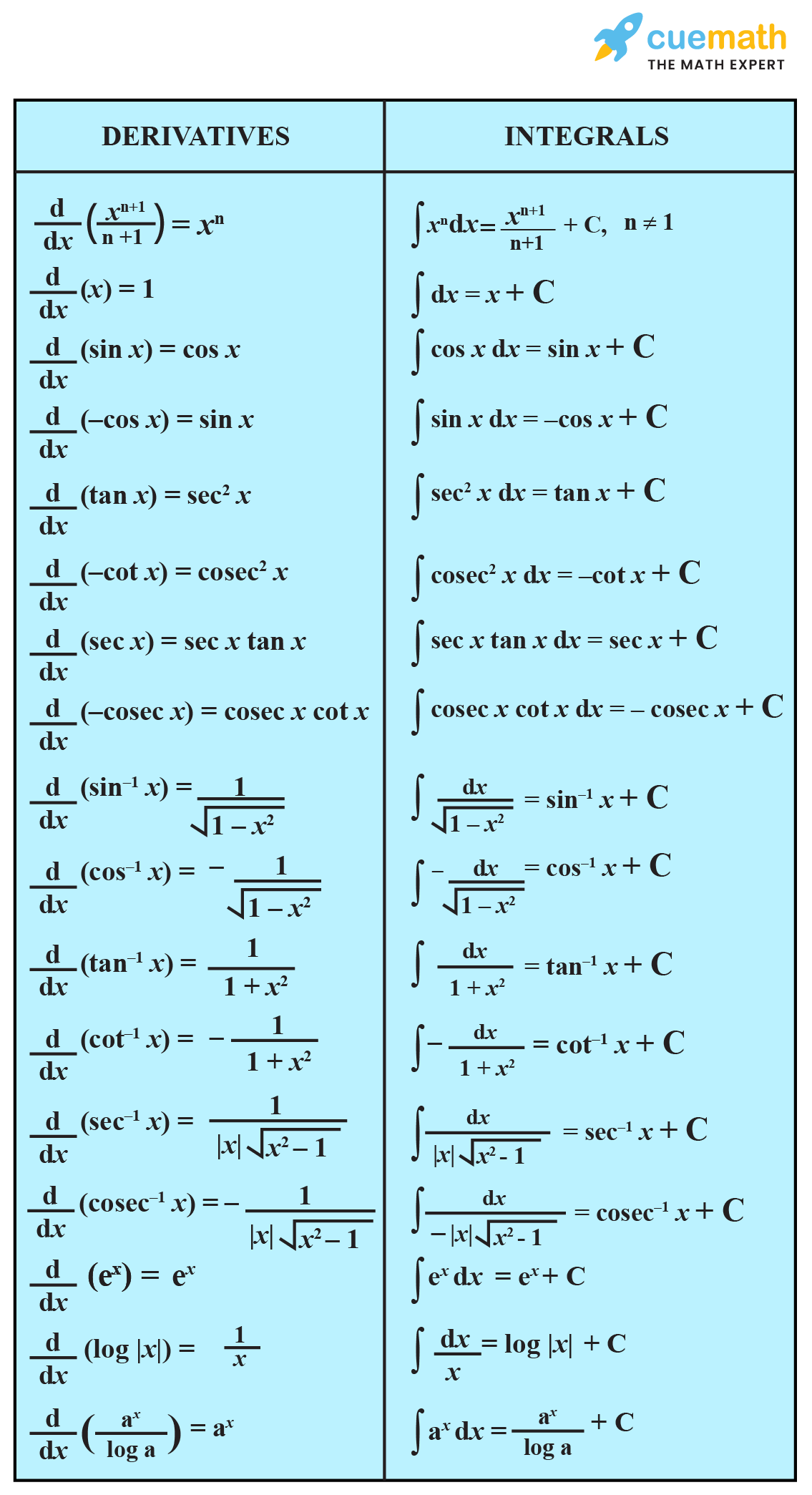More Important Topics
Numbers
Algebra
Geometry
Measurement
Money
Data
Trigonometry
Calculus